# Attraction domain of a stable distribution

(diff) ← Older revision | Latest revision (diff) | Newer revision → (diff)

domain of attraction of a stable distribution

The totality of all distribution functions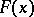such that for a sequence of independent identically-distributed random variables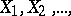with distribution functionand for a suitable choice of constants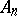and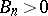,the distribution of the random variable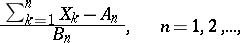(*)

converges weakly, as, to a non-degenerate distribution function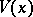, which is necessarily stable.

One of the fundamental problems in the theory of stable laws is the description of domains of attraction of stable laws. Thus, for the normal distribution, A.Ya. Khinchin, W. Feller and P. Lévy established in 1935 thatbelongs to the domain of attraction of a normal law if and only if, as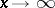,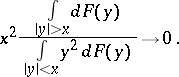Later B.V. Gnedenko and W. Doeblin (1940) gave a description of the domain of attraction of a stable law with exponent,:belongs to the domain of attraction of a non-degenerate stable lawwith exponentif and only if:for some, determined by, andfor each constant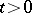. Restriction on the behaviour of the normalizing coefficients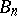,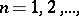leads to narrower classes of distribution functions for which the convergence in distribution (*) holds. The set of distribution functionsfor which (*) converges weakly, for a suitable choice of,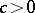and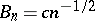,to a stable distribution functionwith exponent, is called the normal domain of attraction for. The normal domain of attraction of a normal distribution coincides with the set of non-degenerate distributions with a finite variance.

The normal domain of attraction of a non-degenerate stable distribution functionwith exponent() is formed by the functionsfor which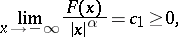exist and are finite, where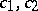are determined by.

How to Cite This Entry:
Attraction domain of a stable distribution. Encyclopedia of Mathematics. URL: http://encyclopediaofmath.org/index.php?title=Attraction_domain_of_a_stable_distribution&oldid=11581
This article was adapted from an original article by B.A. Rogozin (originator), which appeared in Encyclopedia of Mathematics - ISBN 1402006098. See original article